# Design and Application of an Adaptive Slow Feature Extraction Algorithm for Natural Images Based on Visual Invariance

Design and Application of an Adaptive Slow Feature Extraction Algorithm for Natural Images Based on Visual Invariance
Department of Mathematics and Computer Science, Hebei Normal University for Nationalities, Chengde 067000, China

Corresponding Author Email:
zhaoyanming008@163.com
Page:
209-216
|
DOI:
https://doi.org/10.18280/ts.360302
3 March 2019
|
Accepted:
6 May 2019
|
Published:
1 September 2019
| Citation

OPEN ACCESS

Abstract:

The invariance of complex visual information in natural images is not considered in existing slow feature (SF) extraction algorithm. To solve the problem, this paper designs an adaptive SF extraction algorithm for natural images based on visual invariance. Firstly, the principal component analysis (PCA) was improved by the topologically independent component analysis (TICA), aiming to adaptively extract the invariance features of complex visual information in natural images. Next, the Markov chain Monte Carlo (MCMC) algorithm and the visual smoothness theory were combined to solve two defects of the conventional slow feature analysis (SFA): the nonlinear expansion algorithm has nothing to do with the visual invariance of the natural images; the sampling algorithm causes the loss of key visual information. Finally, the probability classification was introduced to our algorithm. The experimental results show that our algorithm achieved higher recognition rate and lower computing complexity than conventional algorithms, and exhibited strong robustness and geometric invariance.

Keywords:

invariant, slow feature (SF), visual computing, receptive field, topology

1. Introduction

The invariant is the component changing at the slowest rate within a fast-changing signal in the time domain. The presence of such a component indicates that the signal source has some inherent properties and that the properties have stable relationships. Therefore, the invariant can be regarded as an abstract invariant information in the high-level expression of high-frequency input signal. The analysis based on invariant learning, a.k.a. slow feature analysis (SFA), can extract the slowly varying properties of the signal source. The research development on slow features (SFs) can be divided into three phases.

The first phase saw the formation of the SF theories and algorithms. In 1989, Hinton  proposed the basic concept, theories and assumptions of the SF, and established the preliminary theory on slow feature extraction. In 1991, Földiák  analyzed the SFs based on online learning theory. Wiskott and Sejnowski relied on transitive closure algorithm to explore the SF algorithms and theories, respectively in 1989 and 2002. In 2002, Wiskott and Sejnowski  put forward the SFA, a milestone in the SF research. In 2007, Franzius et al.  examined the properties of invariant on brain cells in the hippocampus, laying the physiological basis for invariant learning. Sprekeler and Wiskott  developed the cosine optimization form of slowly changing features, ensuring that the SFA can converge to the global optimal solution in the considered function space. In 2008, Franzius et al.  successfully used the SFA to extract and identify the invariant features of the position and rotation angle of cartoon fish, an evidence to the SFA’s ability to extract classification information. The above are the preliminary results on the analysis, algorithms and physiological theory of the SF, which basically prove the excellence of the SFA in feature extraction and recognition.

In the second phase, the SFA was improved and optimized. Inspired by divide-and-conquer strategy, Kompella et al.  presents a hierarchical SFA to reduce the computing complexity. However, much information is lost due to the hierarchical structure, weakening the algorithm’s ability to acquire enough global optimal features. To solve the problem, Porcal et al.  develops the SFA based on graph theory (GSFA), which trains the SFA with the complex structure of the training graph, and extracts features according to the graph structure.

In the third phase, great progress was made in the application of the SFA. With the rapid development of the SF theories and algorithms, the SFA has been extensively applied in such field as human behavior recognition [9-11], text recognition , path tracking [13-15], blind source separation [16, 17], 3D feature extraction [7, 18] and receptive field learning of complex cells .

So far, much progress has been made in the theories and application of the SFA. Nonetheless, there are several defects with the conventional SFA: (1) The algorithm only extracts the SF, without capturing the slowly varying topological relationship between these features; (2) The chromatographic features of natural light or natural materials are extracted by the principal component analysis (PCA), which cannot obtain the invariant visual features adaptively according to the complex visual features contained in natural images; (3) The SFs are expanded by the nonlinear expansion algorithm based on polynomial product, but this algorithm cannot expand the invariant features adaptively according to the complex visual features of the target image.

To overcome these defects, this paper designs and implements an adaptive SF extraction algorithm for natural images based on visual invariance. Firstly, the visual SFs of natural images were divided into slowly varying information (SVI) and SVI-containing slowly varying topology graph (SVTG). The two types of the SFs were extracted from the target natural image by topologically independent component analysis (TICA) , solving the second defect of the conventional SFA. In this way, the invariant features can be extracted adaptively from the complex visual information in natural images. In addition, the Markov chain Monte Carlo (MCMC) algorithm and the visual smoothness theory were combined to expand the base elements of the TICA and create an overcomplete set of weighted near-orthogonal bases. Finally, the probability classification was introduced to our algorithm.

2. Algorithm Design

Natural images are collected by sensors, and contain features that slowly evolve with the natural environment . The slow evolution is similar to the visual structure and functions of humans. Inspired by the theories on the selectivity and smoothness of human vision, the SFs of the complex visual information in natural images could be divided into the SVI and the SVTG. The SVI represents the complex visual invariance information within natural images, while the SVTG, consisting of the SVI topology in the visual space, reveals the visual space distribution of the SVI, and thus boasts good SFs.

In the same scene, the natural images captured by cameras with the same parameters both reflect the evolution of the natural environment, and the concurrent slow variation of the visual space. Therefore, the shooting effects of different cameras can be unified through image processing.

In a natural image, the SFs of the complex visual information can be defined as:

SF={SVI, SVTG}      (1)

As shown in formula (1), the SFs of the natural image in complex visual space contain two SF sets, namely, the SVI and the SVTG. Among them, the SVI represents the complex visual invariance information within the natural image, and the SVTG describes the SVI topology in the visual space. Together, the two SF sets can effectively depict the essence of the natural image.

The conventional SFA extracts the SFs with the PCA. By the PCA, both the natural light and the chromatograms of the light reflected from natural materials can be characterized, and the simple “on-off” visual edge features of natural images can be acquired by cone cells. However, the PCA cannot illustrate the complex visual features of natural images in the visual space. This paper attempts to improve the PCA with the TICA, which can extract the complex visual SVI expressed by the topologically independent components of natural images and interpret the slow variation of complex visual information in natural images from the independence of visual components. Through the improvement, the slow features of a natural image in complex visual space were extracted, forming an SF set. Next, the spatial topological relationships between independent components in the natural image were established and collected to an SVTG set.

In the conventional SFA, the feature space is expanded to higher dimensions by the nonlinear expansion algorithm based on polynomial product. This algorithm expands the SFs in the form of polynomial product. However, the expansion method does not consider the continuous distribution of complex visual features in natural images, failing to reflect the impacts of these features on the extension elements. Drawing on the visual smoothness theory of natural images, the author computed the probability distribution of SVI set elements in the visual space. Then, the MCMC algorithm was initialized based on the distribution, and used to improve the nonlinear expansion algorithm based on polynomial product. The improved algorithm can produce an overcomplete SF set through simultaneous optimization of the SVI and SVTG sets, and optimize the set with near-orthogonal pruning.

Through the above improvements, our algorithm can adaptively extract the visual SFs from natural images based on the invariance of the complex visual information in the images, forming a distinguishable SF set. In this way, the proposed algorithm overcomes the defects of conventional SFA.

3. Algorithm Description

3.1 Improved SF extraction algorithm

The improved SF extraction algorithm is implemented in the following steps:

Step 1. Initialize SF={Φ, Φ}, SVI= Φ and SVTG= Φ.

Step 2. Sequentially select an image I from the image training set. Taking M×N as the sample size, randomly sample max_k sub-images from image I, creating a sub-image training set training_set. Note that M and N are odd numbers smaller than the image size.

Step 3. Execute Step 2 repeatedly until all images in the image training set have been processed, creating a set of sub-image training sets training_set={sub_image: label}, where sub-image is a sub-image training set and label is the label of that sub-image training set.

Step 4. Normalize the set of sub-image training sets.

Step 5. Fit the sub-image elements in the set of sub-image training sets with the Gabor function of the TICA, and generate the SVI of each set SVI={SVI(i): label| i=1, 2, 3, 4…N}, where i is the type of image.

Step 6. Calculate the joint distribution probability of the frequency and direction angle of the Gabor element in SVI(i). On this basis, initialize the MCMC algorithm and use it for nonlinear expansion of SVI(i). Then, perform near-orthogonal pruning over the expanded Gabor function and the Gabor function extracted from the images, using the near-orthogonal criterion R_cross. Remove the elements of the expanded SVI(i) that are not orthogonal to the SVI elements, and thus optimize the SVI set.

The near-orthogonal criterion R_cross can be expressed as:

operation $=\left\{\begin{array}{l}{\text { Pr } \text {une} \text {the element,if }\langle\beta \bullet \beta\rangle \succ \varepsilon} \\ {\text { Reserve the element,if }\langle\alpha, \beta\rangle \leq \varepsilon}\end{array}\right.$ (2)

where, α and β are elements in the expanded SVI set SVI (i); ε is the pruning control coefficient, whose value could be any positive decimal.

Step 7. Define the topology criterion in complex visual space R_complex_visual and use this criterion to convert the SVI into a complex visual invariant feature distribution matrix. In addition, set up the spatial topology matrix SVTG (i) of each image based on the distribution of SVI (i).

$S V I=\left[\begin{array}{ccccc}{g 11} & {g 12} & {g 13} & {\cdots} & {g 1 n} \\ {g 21} & {g 22} & {g 23} & {\cdots} & {g 2 n} \\ {} & {} & {} & {} & {} \\ {} & {} & {} & {} & {} \\ {g k 1} & {g k 2} & {g k 3} & {\cdots} & {g k n}\end{array}\right]$

$\operatorname{SVTG}(i)=\left[\begin{array}{ccccc}{v 11} & {v 12} & {v 13} & {\cdots} & {v 1 n} \\ {v 21} & {v 22} & {v 23} & {\cdots} & {v 2 n} \\ {} & {} & {} & {} \\ {} & {} & {} & {} \\ {v k 1} & {v k 2} & {v k 3} & {\cdots} & {v k n}\end{array}\right]$

where, gij is a Gabor function depicting the invariant complex visual features; vij indicates whether the SVI(i) has invariant complex visual features or not. The SVI is a global matrix generated from the image training set, while the SVTG matrix presents the topological relationship of local invariance in a specific image.

The topology criterion in complex visual space R_complex_visual is explained as follows.

(1) SVI generation rule:

Divide the SVI(i) into K rows by frequency. Each row has p×360 elements, where p is the algorithm resolution. Next, assign the row non-deformation feature to specified positions according to the direction angle of the Gabor function, creating the hierarchical traversal diagram of the invariance of complex visual features SVI.

(2)SVTG generation rule:

The slowly varying topology of the i-th image in the complex visual space can be expressed as:

$S V T G(i)=\left\{\begin{array}{ll}{1} & {\operatorname{gabor}(i, j) \neq 0} \\ {0} & {\operatorname{gabor}(i, j)=0}\end{array}\right.$ (3)

Thus, the invariant complex visual features can be described as SF={SVI, SVTG}.

3.2 Image recognition method

Step 1. Select image I from the image training set. Generate the test set test_set by windowing the training images.

Step 2. Compute the row-by-row convolution of test_set and SVI, according to the distribution of nonzero elements of SVTG(i) in each row of the SVI. If the convolution value is close to 1, assign 1 to the corresponding position of the feature set; otherwise, assign 0 to that position. In this way, generate the feature set feature_set.

Step 3. Compute the Mahalanobis distance similarity between feature_set and SVTG(i). If the similarity is close to 1, then the image belongs to the i-th type.

Step 4. Execute Steps 2~3 repeatedly until all elements in the SVTG have been processed.

4. Experimental Verification and Results Analysis

4.1 Sample collection and processing

A total of 1,000 images were selected from the natural image database INRIA Holidays dataset, forming a set of original images resource_set. Then, random affine transformations were performed on this set. For each image, six new images were created through rotation by less than 10°, 7 were created by translation, and 6 were created by scaling. Overall, 19 new images were obtained from each image in the resource_set. The new images and the new images were combined into the image database for our experiment. The 20,000 images in the database were divided into two parts. The first 15,000 images were grouped into the training set train_set, and the remaining 5,000 were collected into the test set test_set. Each set contains the 1,000 original images. Figure 1 presents some samples from the natural image database.

## 1.jpg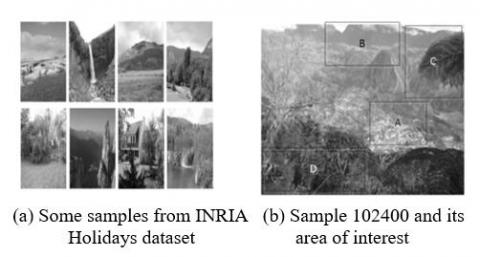Figure 1. Some samples from the natural image database

4.2 Invariance of the number of base elements

The pixels on sample 102400 were collected seven times from the specified areas A, B, C and D, respectively. The number of pixels being collected each time can be defined as 2N (N=7, 8, 9, 10, 11, 12, 13). The four areas are of the size M1×N1=7×7, where M1 and N1 are the number of pixels. Then, the proposed algorithm was adopted to determine the relationship between the number of base elements in complex visual space of the natural image and the number of sample collections.

## 2.png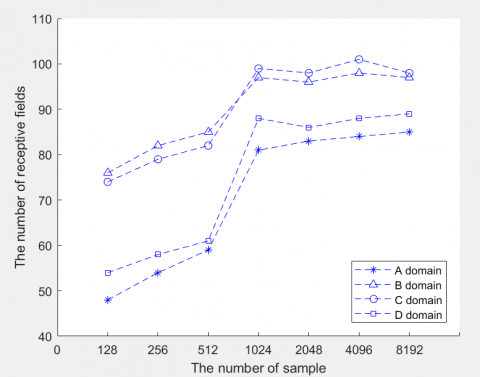Figure 2. Invariance of the number of base elements

As shown in Figure 2, with the increase in the number of sample collections, the number of base elements in complex visual space of the natural image firstly increased and then stabilized, if they are within the identical or similar visual function areas in the natural image. The results demonstrate the slow variation of the number of base elements in complex visual space of the natural image. When the number of samples reached 1,024, the number of base elements in complex visual space of the natural image tended to be stable. Thus, the information loss induced by sampling can be solve by increasing the number of sample collections.

4.3 Invariance of the local topology of base elements

The pixels were collected from sample 102400 by the same method as in subsection 4.2. Then, the proposed algorithm was adopted to determine the relationship between the local topology of base elements and the number of sample collections.

## 3.jpg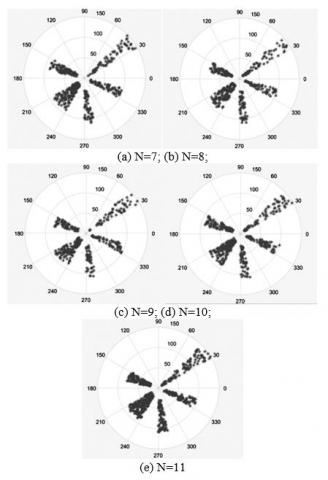Figure 3. Invariance of the local topology of base elements

As shown in Figure 3, the local topology of base elements in complex visual space did not change significantly with the growth in the number of sample collections, if they are of identical or similar frequencies. This means the local topology of base elements in different complex visual spaces is slowly varying, if they belong to the same visual function block; but the number of base elements will increase. When the number of sample collections N reached 10, the number of base elements became stable in the main area generated by the algorithm.

4.4 Invariance of the types and global topology of base elements

The pixels on sample 102400 were collected randomly thirteen times. The number of pixels being collected each time can be defined as 2N (N=5, 6, 7, …, 17). The collection area is of the size M1×N1=7×7. The collected samples were accumulated. Then, the proposed algorithm was adopted to determine the relationship between the number of base element types in the natural image and the number of sample collections, and that between the global topology of base elements and the number of sample collections.

## 4.pngFigure 4. Invariance of the types of base elements

## 51.pnga) The value of frequency is 25.63 HZ

## 52.png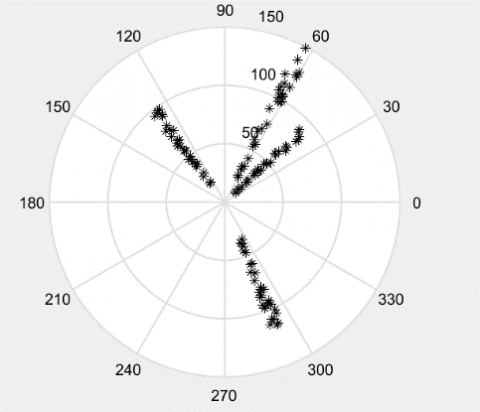b) The value of frequency is 34.56

## 53.pngc) The value of frequency is 41.45 HZ

## 54.pngd) The value of frequency is 60.19HZ

Figure 5. Invariance of global topology of base elements

As shown in Figure 4, with the increase in the number of sample collections, the number of base elements in complex visual space of the natural image firstly increased and then stabilized. After the samples had been collected six or more times, the number of perceptive fields in the set stabilized at around 30. These trends could be explained by following: Under a limited number of sample collections, some pixels may be collected from the same area(s). Then, the collected samples cannot represent the visual blocks of the entire image, causing the oscillation of complex visual blocks. By contrast, repeated sample collections can acquire the complex visual blocks of the natural image stably. Due to the slow variation in the number of visual function blocks, the number of base element types in the complex visual space will remain unchanged.

As shown in Figure 5, with the increase in the number of sample collections, the topology of different base elements of the natural image became increasingly stable, under all frequencies and in all direction angles. This proves the slow variation in the global topology of base elements in complex visual space of the natural image.

4.5 Invariance of visual structure SFs

The pixels on natural image P001 were collected randomly one hundred times. Each time, 1,024 pixels were collected. The collection area is of the size M1×N1=7×7. The collected samples were accumulated. Then, the proposed algorithm was adopted to analyze the visual structure SFs of the four main distribution planes of the natural image.

## 6.jpgFigure 6. Invariance of visual structure SFs

As shown in Figure 6, the base elements in complex visual space, which make up the visual structure SFs, were invariant in terms of their types, numbers, local topology and global topology. This shows the slow variation in the visual structure of the natural image.

To sum up, the microscopic analysis on the complex vision of natural images shows that the types and numbers of the base elements in complex visual space are invariant, so are the local and global topologies of these base elements.

4.6 Classification performance of our algorithm

A total of 1,024 samples were collected from the test set test_set and the training set train_set, respectively, with the collection areas of the size M1×N1=7×7. Then, our algorithm was adopted to compute the intra-class distribution and inter-class distribution.

## 7.jpg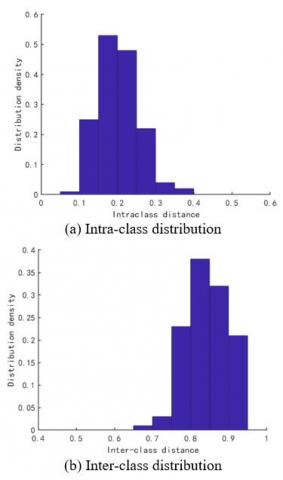Figure 7. The classification performance of our algorithm

As shown in Figure 7, when the threshold was 0.35, our algorithm correctly recognized 99.96 % of all samples. Both false acceptance rate (FAR) and false rejection rate (FRR) were smaller than 3 ‰. The results prove the strong classification ability of our algorithm.

4.7 Performance comparison

The samples were collected randomly one hundred times from the test set test_set and the training set train_set, respectively. Each time, 1,024 samples were collected. The collection area is of the size M1×N1=7×7. Then, the proposed algorithm, the SFA , the GSFA  and the TICA  were separately applied to recognize the samples. The comparative results are plotted as Figure 8.

As shown in Figure 8, our algorithm outperformed the three contrastive algorithms in classification ability. The advantage increased with the number of sample collections. The recognition rate of our algorithm finally stabilized at 99.89 %.

## 8.pngFigure 8. Comparison of recognition performance

4.8 Noise immunity

The samples were collected by the same method as in subsection 4.7. Then, the author analyzed the effects of sampling window size, noise type and noise intensity on our algorithm.

As shown in Figure 9, when the noise type remained the same, the recognition rate of our algorithm declined with the growth in noise intensity; when the noise intensity remained constant, the recognition rate of our algorithm plunged if the noise type was similar to the type of the receptive filed; the smaller the sampling window, the greater the impacts of noise on the recognition rate; the number of sampling windows has no significant effect on the recognition rate. Below is a detailed analysis on the effect of each type of noise.

## 91.png(a) Effects of sampling window size

## 92.png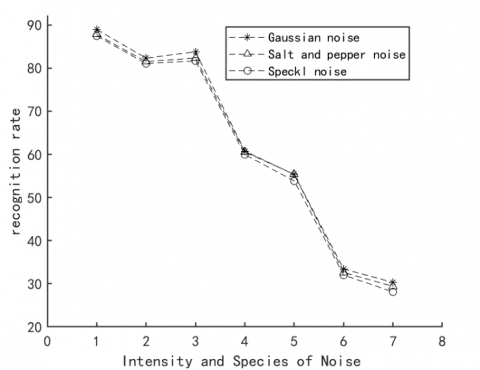(b) Effects of noise type and noise intensity

Figure 9. Effects of sampling window size, noise type and noise intensity on our algorithm

(1) Gaussian noise has an obvious “on-off” selectivity, and its function expression is a subset of the Gabor function. Thus, the increase of Gaussian noise will change the attributes and features of the base function for local visual space, such that the extracted Gabor function cannot describe the essence of the original natural image. However, Gaussian noise has little impact on topology.

(2) Salt-and-pepper noise carries the features of black and white point noise, and has a small amount of visual “on-off” selectivity. This type of noise exerts a major impact on the extraction of local features, and greatly affects the extraction of regional Gabor function. The impact is particularly serious if the sampling window is relatively small. Salt-and-pepper noise has a greater effect on local and global topologies than Gaussian noise.

(3) Compared with the above two noises, the speckle noise has a large single structure. Each speckle noise can form an independent visual Gabor function, and produce independent visual selectivity, while destroying the visual selectivity and blocks of the original natural images. Hence, the receptive field features extracted by our algorithm cannot characterize the exact visual features of the original images.

In general, under the same noise intensity, speckle noise has the greatest impact on the recognition rate of our algorithm, followed in turn by salt-and-pepper noise and Gaussian noise.

4.9 Geometric invariance

The samples were collected by the same method as in subsection 4.7. Then, the relationship between geometric invariance and the performance of our algorithm was determined.

Table 1. Relationship between geometric invariance and algorithm performance

 rotation Angle of Rotation 1 3 7 11 13 19 21 Recognition rate 99.76 97.92 92.03 84.18 80.21 76.46 69.21 translation the number of pixels 3 5 7 11 21 23 35 Recognition rate 99.34 98.51 99.31 98.38 97.67 98.89 99.15 scale the factor of scale 1 2 3 1/2 1/4 1/8 1/16 Recognition rate 99.51 98.81 96.11 98.61 97.81 87.26 84.21

As shown in Table 1, the performance of our algorithm was immune to a small rotation angle, and slightly affected by a large rotation angle. When the number of samples remained the same, the changing translation distance had no or slight impact on the algorithm performance, revealing the translation invariance of our algorithm. When the number of sample collections remained unchanged, the recognition rate of our algorithm gradually decreased with the growth in the scale. Hence, the scaling has only a little impact on the set invariance of our algorithm.

5. Conclusions

This paper puts forward an adaptive SF extraction algorithm for natural images based on visual invariance. Firstly, the invariance of complex visual features in natural images was highlighted, which is reflected by the invariance of complex visual information and the invariance of the topology of the base elements forming the information in the visual space. On this basis, the traditional SF extraction algorithm was improved, and then applied to extract the SFs from natural images, covering the type of complex visual components, the number of elements in each class, the intra-class topology and the inter-class topology. In addition, the MCMC algorithm and the visual smoothness theory were introduced to create an overcomplete set of SFs, solving the computing complexity of the nonlinear expansion algorithm of the traditional SFA. The overcomplete set was optimized by the near-orthogonal pruning method, yielding the optimized near-orthogonal overcomplete SFs. The experimental results show that the complex visual information of natural images does have the SFs, and our algorithm outperformed the other algorithms in recognition rate, computing complexity and feasibility. In addition, our algorithm was found to have strong noise-resistance and set invariance under priority conditions.

The future research will further improve our algorithm from three aspects: (1) explore the algorithm performance on large sample sets; (2) employ the algorithm in other image applications; (3) explore the deep structure and parallel application of the algorithm from the angle of deep visual network.

Acknowledgment

This work is supported by the Social science foundation of Hebei province of china (Grant numbers: HB18TJ004), Science and technology planning project of Hebei Province of China (Grant numbers: 15210135), National Science and Technology Infrastructure Program (Grant numbers: 2015BAH43F00).

References

 Hinton, G.E. (1989). Connectionist learning procedures. Artificial Intelligence, 40(1-3): 185-234. https://doi.org/10.1016/0004-3702(89)90049-0

 Földiák, P. (1991). Learning invariance from transformation sequences. Neural Computation, 3(2): 194-200. https://doi.org/10.1162/neco.1991.3.2.194

 Wiskott, L., Sejnowski, T.J. (2002). Slow feature analysis: Unsupervised learning of invariances. Neural Computation, 14(4): 715-770. https://doi.org/10.1162/089976602317318938

 Franzius, M., Sprekeler, H., Wiskott, L. (2007). Slowness and sparseness lead to place, head-direction, and spatial-view cells. PLoS Computational Biology, 3(8): e166. https://doi.org/10.1371/journal.pcbi.0030166

 Sprekeler, H., Wiskott, L. (2011). A theory of slow feature analysis for transformation-based input signals with an application to complex cells. Neural Computation, 23(2): 303-335. https://doi.org/10.1162/neco_a_00072

 Franzius, M., Wilbert, N., Wiskott, L. (2008). Invariant object recognition with slow feature analysis. Artificial Neural Networks, ICANN 2008, 18th International Conference,Prague,Czech Republic, Proceedings, Part I. Springer-Verlag, 2008.

 Kompella, V.R., Luciw, M., Schmidhuber, J. (2011). Incremental slow feature analysis. Artificial Intelligence Not Elsewhere Classified, 1354-1359. https://doi.org/10.5591/978-1-57735-516-8/IJCAI11-229

 Porcal, P., Dillon, P.J., Molot, L.A. (2013). How to solve classification and regression problems on high-dimensional data with a supervised extension of slow feature analysis. Journal of Machine Learning Research, 14(18): 3683-3719. https://doi.org/10.1049/iet-cta.2013.0741

 Koch, P., Konen, W., Hein, K. (2010). Gesture recognition on few training data using Slow Feature Analysis and parametric bootstrap. Neural Networks (IJCNN), The 2010 International Joint Conference on IEEE, 2010. https://doi.org/10.1109/IJCNN.2010.5596842

 Zhang, Z., Tao, D.C. (2012). Slow feature analysis for human action recognition. IEEE Trans Pattern Anal Mach Intell, 34(3): 436-450. https://doi.org/10.1109/TPAMI.2011.157

 Chen, T.T., Ruan, Q.Q., An, G.Y. (2015). Slow feature extraction algorithm of human actions in video. CAAI Transactions on Intelligent Systems, 10(3): 381-386.

 Berkers, C.R., Verdoes, M., Lichtman, E., Fiebiger, E., Kessler, B.M., Anderson, K.C., Ploegh, H.L., Ovaa, H., Galardy, P.J. (2005). Activity probe for in vivo profiling of the specificity of proteasome inhibitor bortezomib. Nature Methods, 2(5): 357-362. https://doi.org/10.1038/nmeth759

 Kühnl, T., Kummert, F., Fritsch, J. (2011). Monocular road segmentation using slow feature analysis. 2011 IEEE Intelligent Vehicles Symposium (IV). https://doi.org/10.1109/IVS.2011.5940416

 Zhang, Y., Yang, Y.X., He, H.H. (2014). DOA estimation method performance study based on slowness feature analysis. Computer Simulation, 2014(5): 179-182.

 Hao, T., Wang, Q., Wu, D., Sun, J.S. (2018). Multiple person tracking based on slow feature analysis. Multimedia Tools and Applications, 77(3): 3623-3637. https://doi.org/10.1007/s11042-017-5218-4

 Ma, K.J., Han, Y.J., Tao, Q., Wang, J. (2011). Kernel-based slow feature analysis. Pattern Recognition and Artificial Intelligence, 2011(2): 153-159.

 He, H.H., Li, G.H., Yao, Q.S., He, X.K., Shi, C.X. (2014). Blind source separation of underwater acoustic signals by using slowness feature analysis. Technical Acoustics, 2016(3): 270-274.

 Nie, W.Z., Liu, A.N., Su, Y.T., Wei, S. (2017). Multi-angle view of the feature extraction based on slow feature analysis. Neurocomputing, 252. https://doi.org/10.1016/j.neucom.2016.01.125

 Zhao, Y.M., Fang, J., Ji, S.J. (2015). Feature extraction algorithm based on complex visual information of natural image and application. Computer Applications and Software, 32(11): 200-205. https://doi.org/10.3969/j.issn.1000-386x.2015.11.047